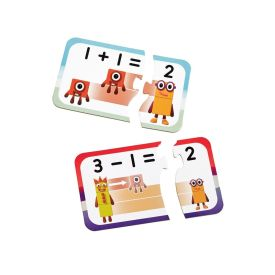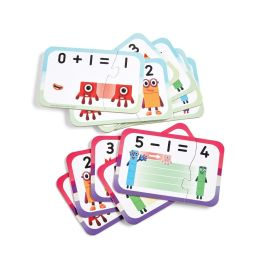• New
••••# NumberBlocks Adding and Subtracting Puzzle

95402

This first Numberblocks puzzle helps little ones learn basic addition and subtraction skills with their friends the Numberblocks. There are 10 addition and 10 subtraction puzzles featuring Zero to Ten. Each Numberblocks addition puzzle and Numberblocks subtraction puzzle has 2 pieces. Match the simple maths question to the correct answer piece to complete the puzzle. Each clever puzzle fits together in a unique way so there’s only one right answer. This gives children a sense of achievement as they figure out the answers themselves.

€18.50
Tax included Delivery within 3-4 days

With this Numberblocks jigsaw puzzle, children learn what happens when they add or subtract one.

There are 10 addition puzzles and 10 subtraction puzzles featuring Numberblocks Zero to Ten.

Each of these clever puzzles fits together in a unique way, which means there’s only one correct answer. This gives children a sense of achievement as they figure out the answers themselves.

Made from durable card, the puzzles pieces store in the reusable box.

Includes 20 x 2-piece puzzles.

Completed puzzles measure 15cm L x 10cm W.

95402